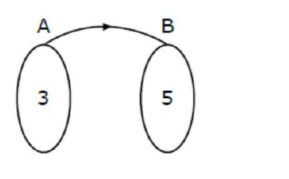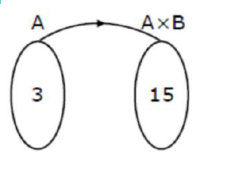# Let x denote the total number of one-one functions from

Question:

Let $x$ denote the total number of one-one functions from a set $A$ with 3 elements to a set $B$ with 5 elements and $y$ denote the total number of one-one functions from the set $A$ to the set $A \times B$. Then:

1. (1) $y=273 x$

2. (2) $2 y=91 x$

3. (3) $y=91 x$

4. (4) $2 y=273 x$

Correct Option: , 2

Solution:

Number of elements in $A=3$

Number of elements in $\mathrm{B}=5$ Number of elements in $A \times B=15$Number of one-one function $x=5 \times 4 \times 3$

$x=60$Number of one-one function $y=15 \times 14 \times 13$

$y=15 \times 4 \times \frac{14}{4} \times 13$

$y=60 \times \frac{7}{2} \times 13$

$2 y=(13)(7 x)$

$2 y=91 x$